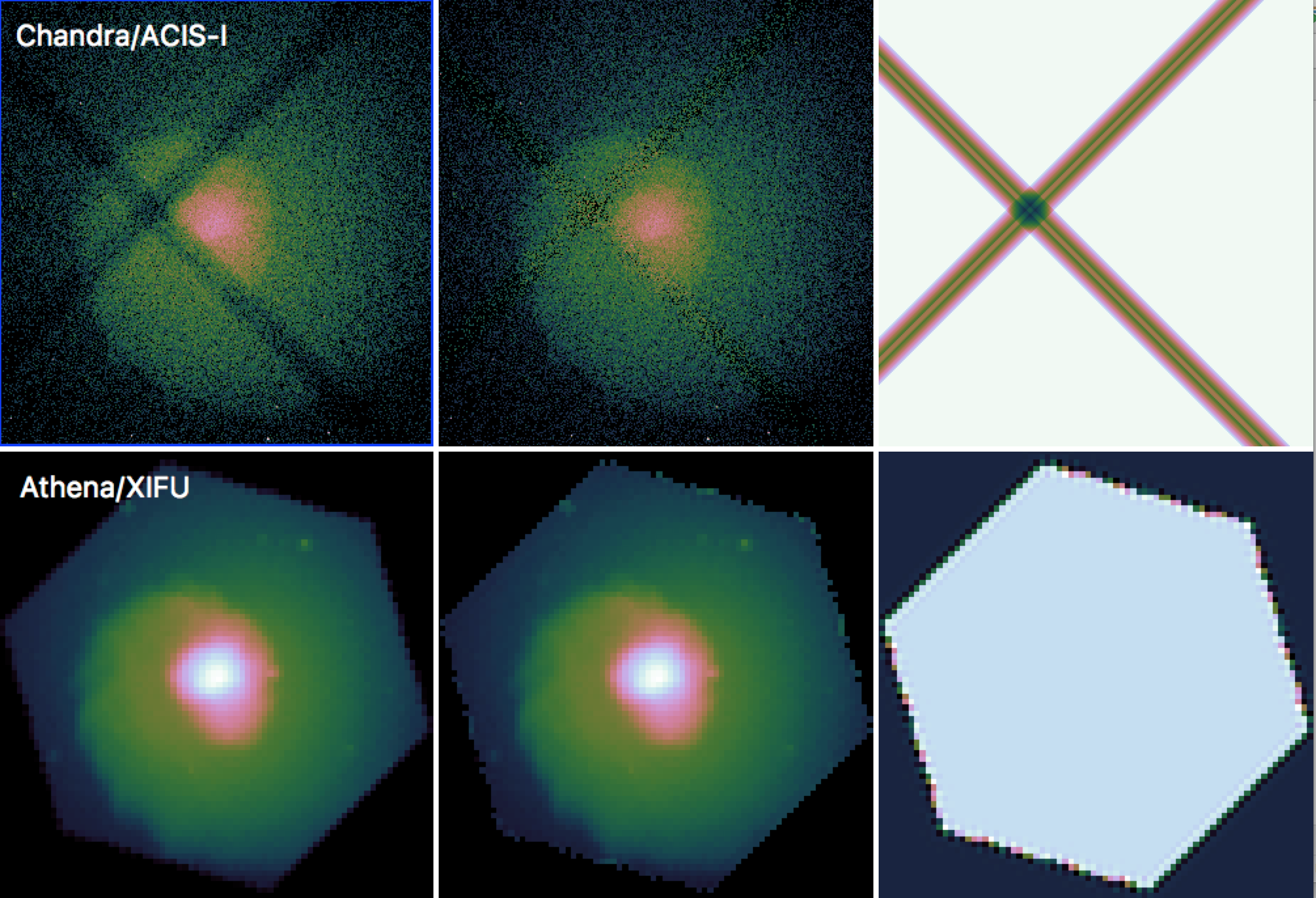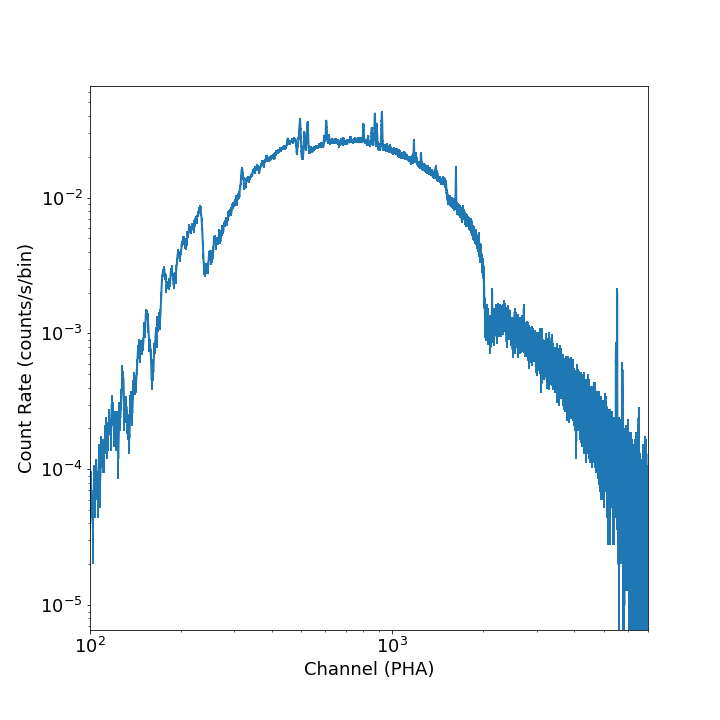# Event File Tools¶

This section documents some helpful tools to take event files produced by the SOXS instrument simulator and make derivative products from them.

## filter_events¶

The filter_events() function is used to filter event files on event position or energy, and make a new event file. This may be useful if you want to analyze a subset of the data only. To filter out events based on energy:

# Filter out everything less than 0.5 keV
soxs.filter_events("evt.fits", "evt_lt0.5.fits", emin=0.5, overwrite=True)

# Filter out everything greater than 2.0 keV
soxs.filter_events("evt.fits", "evt_gt2.0.fits", emin=2.0, overwrite=True)

# Filter out everything except within the 0.1-3.0 eV band
soxs.filter_events("evt.fits", "evt_gt2.0.fits", emin=0.1, emax=3.0, overwrite=True)


To filter out events based on position, we use a region file from ds9, CIAO, etc.:

soxs.filter_events("evt.fits", "evt_circle.fits", region="circle.reg",
overwrite=True)


Alternatively, you can use a region string:

reg = '# Region file format: DS9\nimage\ncircle(147.10,254.17,3.1) # color=green\n'
soxs.filter_events("evt.fits", "evt_circle.fits", region=reg,
overwrite=True)


Energy and region filtering can be combined:

soxs.filter_events("evt.fits", "evt_circle.fits", region="circle.reg",
overwrite=True, emin=0.1, emax=0.5)


To filter on a time range:

soxs.filter_events("evt.fits", "evt_shorter.fits", emin=0.5, emax=7.0, tmin=0.0,
tmax=(200.0, "ks"))


## make_exposure_map¶

Exposure maps are maps of exposure for a given X-ray energy or range of energies, giving the effective exposure as a function of position on the sky. Uneven exposure may occur because of spacecraft dithering, chip gaps, or differences in effective area at different points on a chip (though the latter effect is currently not simulated in SOXS). For a more thorough explanation of exposure maps, see Introduction to Exposure Maps.

make_exposure_map() creates an exposure map for SOXS event files. This may be needed particularly if you have a simulation with dither and are concerned about effects near the edges of chips, but it will work for any event file produced by SOXS. This function determines the effective area for a given input energy or range of energies weighted by a spectrum, and then uses the aspect solution (the RA and Dec of the spacecraft pointing as a function of time) to create a histogram of aspect to determine how much time a given detector coordinate spent on a given sky location. These pieces of information are then used to produce a map of total exposure for the observation in sky coordinates. Exposure maps can be “reblocked” to a lower resolution, and this will typically speed up the computation of the exposure map.

To make an exposure map with the default parameters using an energy of 4.0 keV:

import soxs
soxs.make_exposure_map("my_evt.fits", "my_expmap.fits", 4.0, overwrite=True)


If you have an idea of what the spectrum of the object is, you can alternatively use an energy range and the spectrum itself as a set of “weights” to make a weighted exposure map. This example uses a Spectrum object spec:

import soxs

energy = spec.emid.value
weights = spec.flux.value

soxs.make_exposure_map("my_evt.fits", "my_expmap.fits", energy, weights=weights,
overwrite=True)


By default, exposure maps are computed in units of $$\rm{cm^2} \times \rm{s}$$ and then divided by the exposure time to get a map in units of $$\rm{cm}^2$$. To turn off this normalization and get a map in units of $$\rm{cm^2} \times \rm{s}$$, set normalize=False:

import soxs
soxs.make_exposure_map("my_evt.fits", "my_expmap.fits", 4.0,
overwrite=True, normalize=False)


To write the aspect solution to a file, set the asol_file keyword to the file you want to use:

import soxs
soxs.make_exposure_map("my_evt.fits", "my_expmap.fits", 4.0,
overwrite=True, asol_file="my_asol.fits")


By default, the aspect is binned into a histogram of 16x16 bins covering the range of the aspect solution pattern. To change this binning, use the nhistx and nhisty keywords:

import soxs
soxs.make_exposure_map("my_evt.fits", "my_expmap.fits", 4.0,
overwrite=True, nhistx=32, nhisty=32)


To create an exposure map with pixels 4 times larger on a side, set the reblock parameter to 4:

import soxs
soxs.make_exposure_map("my_evt.fits", "my_expmap.fits", 4.0,
overwrite=True, reblock=4)


Examples of images and exposure maps for a simulation of a galaxy cluster for Chandra/ACIS-I and Athena/XIFU are shown in Figure 1.Figure 1: Images (left), exposure-corrected images (center) and exposure maps (right) for mock observations of a galaxy cluster for Chandra/ACIS-I (top) and Athena/XIFU (bottom), simulated using SOXS.¶

Warning

The make_exposure_map() tool only produces exposure maps for event files produced by SOXS, and this is the only tool that should be used for this purpose for event files produced by SOXS.

## write_image¶

write_image() bins up events into an image according to the coordinate system inherent in the event file and writes the image to a FITS file. Images of sky, detector, or chip coordinates can be written. You can also restrict events within a particular energy range or time range to be written to the file.

To write an image in sky coordinates:

from soxs import write_image
# Energy bounds are in keV
write_image("my_evt.fits", "my_sky_img.fits", emin=0.5, emax=7.0)


Or in detector coordinates:

write_image("my_evt.fits", "my_det_img.fits", coord_type='det', emin=0.5, emax=7.0)


To filter on a time range:

write_image("my_evt.fits", "my_sky_img.fits", emin=0.5, emax=7.0, tmin=0.0,
tmax=(200.0, "ks"))


To filter on multiple energy bands, use a list of tuples of minimum, maximum energy of each band (in keV) supplied to the bands keyword (this is an alternative to emin and emax, and if present will override them:

write_image("my_evt.fits", "my_sky_img.fits", bands=[(0.5, 2.0), (4.0, 6.0)])


To supply an exposure map produced by make_exposure_map() to make a flux image:

write_image("my_evt.fits", "my_sky_img.fits", coord_type='sky', emin=0.5, emax=7.0,
expmap_file="my_expmap.fits")


To bin at a pixel size 4 times larger than the native pixel size, set reblock to 4:

write_image("my_evt.fits", "my_sky_img.fits", coord_type='sky', emin=0.5, emax=7.0,
expmap_file="my_expmap.fits", reblock=4)


Note that if you set reblock and supply an exposure map, it must have been made with the same value of reblock.

This image can then be viewed in ds9 or APLpy.

## make_image¶

make_image() is almost identical to write_image(), but it does not request an output filename. Instead, it bins up events into an image according to the coordinate system inherent in the event file and returns an ImageHDU object. Otherwise, the rest of the arguments to make_image() are the same as to write_image().

## write_radial_profile¶

write_radial_profile() bins up events into an radial profile defined by source center, a minimum radius, a maximum radius, and a number of bins. One can restrict the events that are binned by a specific energy band. An example execution:

from soxs import write_radial_profile
ctr = [30.0, 45.0] # by default the center is in celestial coordinates
rmin = 0.0 # arcseconds
rmax = 100.0 # arcseconds
nbins = 100 # number of bins
emin = 0.5 # keV
emax = 2.0 # keV
rmax, nbins, emin=emin, emax=emax, overwrite=True)


If one wants to specify a center in physical pixel coordinates, you can use the same execution but set the ctr_type keyword to “physical” and use physical pixel coordinates as the ctr argument:

from soxs import write_radial_profile
ctr = [2048.5, 2048.5] # by default the center is in celestial coordinates
rmin = 0.0 # arcseconds
rmax = 100.0 # arcseconds
nbins = 100 # number of bins
emin = 0.5 # keV
emax = 2.0 # keV
rmax, nbins, ctr_type="physical", emin=emin, emax=emax,
overwrite=True)


If one wants to compute flux-based quantities for the radial profile (such as surface flux), supply an exposure map produced by make_exposure_map():

write_radial_profile("my_evt.fits", "my_radial_profile.fits", ctr, rmin,
rmax, nbins, ctr_type="physical", emin=emin, emax=emax,
expmap_file="my_expmap.fits", overwrite=True)


A cookbook example showing how to extract a radial profile is shown in Radial Profile.

## write_spectrum¶

write_spectrum() bins up events into a spectrum and writes the spectrum to a FITS file:

from soxs import write_spectrum
write_spectrum("my_evt.fits", "my_spec.pha", overwrite=True)


This spectrum file can be read and fit with standard X-ray analysis software such as XSPEC, ISIS, and Sherpa.

To filter out events based on position for the spectrum, we can use a region file from ds9, CIAO, etc.:

write_spectrum("my_evt.fits", "my_spec.pha", region="circle.reg",
overwrite=True)


Alternatively, you can use a region string:

reg = '# Region file format: DS9\nimage\ncircle(147.10,254.17,3.1) # color=green\n'
write_spectrum("my_evt.fits", "my_spec.pha", region=reg, overwrite=True)


Filtering on a time or energy range is also possible:

write_spectrum("my_evt.fits", "my_spec.pha", emin=0.5, emax=2.0,
tmin=0.0, tmax=(0.5, "Ms"), overwrite=True)


## plot_spectrum¶

plot_spectrum() reads a spectrum stored in a FITS table file and makes a Matplotlib plot. There are a number of options for customizing the plot in the call to plot_spectrum(), but the method also returns a tuple of the Figure and the Axes objects to allow for further customization. This example opens up a spectrum file and plots it between 0.5 and 7.0 keV:

from soxs import plot_spectrum
plot_spectrum("evt.pha", xmin=0.5, xmax=7.0)If one wanted to plot the same spectrum in channel space instead of energy space, you would set plot_energy=False:

from soxs import plot_spectrum
plot_spectrum("evt.pha", plot_energy=False, xmin=300, xmax=7000)where in that case the x-axis is now in channel space, so xmin and xmax had to be set accordingly.

To bin the spectrum in energy bins of your choice, generate a set of bin edges and pass them to plot_spectrum() using the ebins argument:

from soxs import plot_spectrum
import numpy as np
ebins = np.linspace(0.5, 7.0, 101)
plot_spectrum("evt.pha", ebins=ebins, xmin=0.5, xmax=7.0)


For other customizations, consult the plot_spectrum() API.

## plot_image¶

The plot_image() function allows one to plot an image from a FITS file. Several examples of this are shown in the following cookbook recipes:

For the full range of customizations, consult the plot_image() API.

## merge_event_files¶

soxs.events.merge_event_files() can be used to merge several event files together. This may be useful if you want to merge a source file with a background file created later, for example.

soxs.merge_event_files(["src1_evt.fits", "src2_evt.fits", "bkg_evt.fits"],
"merged_evt.fits", overwrite=True)


## fill_regions¶

fill_regions() can be used to fill in regions with background counts in an image which has had bright sources removed by a tool such as wavdetect. In addition to the file containing the image to be filled and the name of the new file to be written, the user supplies a region or list of regions to fill in (region strings, ds9 region files, Region objects, or a Regions object), and bkg_value, which is either a single floating-point value for the number of background counts, a single region from which to get this number, or a list of regions (of the same number as the source list of regions) to obtain this number from. By default, bkg_value number for each region will serve as the mean of a Poisson distribution from which random numbers of counts will be drawn for each pixel in each region to be filled. If a region or regions are supplied for bkg_value, by default the mean number of counts from each region will be used to calculate this value, though it is also possible to use the median value.

Here is an example where we want to fill a single region with the counts where the mean from is taken another region:

soxs.fill_regions("holed_img.fits", "filled_img.fits", "src.reg", "bkg.reg",
overwrite=True)


Here is the same example, except that we use the median number of counts within "bkg.reg" to determine the mean of the Poisson distribution to fill with:

soxs.fill_regions("holed_img.fits", "filled_img.fits", "src.reg", "bkg.reg",
median=True, overwrite=True)


Here is an example where we fill the hole supplying a single number for the mean of the Poisson distribution:

soxs.fill_regions("holed_img.fits", "filled_img.fits", "src.reg", 10.0,
overwrite=True)